# The Big Mac Index

The Big Mac index, published by The Economist from 2000 to 2018.

THE Big Mac index was invented by The Economist in 1986 as a lighthearted guide to whether currencies are at their "correct" level. It is based on the theory of purchasing-power parity (PPP), the notion that in the long run exchange rates should move towards the rate that would equalise the prices of an identical basket of goods and services (in this case, a burger) in any two countries.

## Examples

### Basic Examples

Retrieve the resource:

 In:=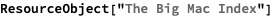Out=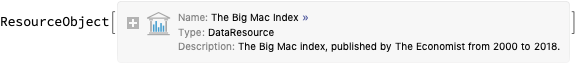Retrieve the default content:

 In:=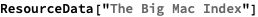Out=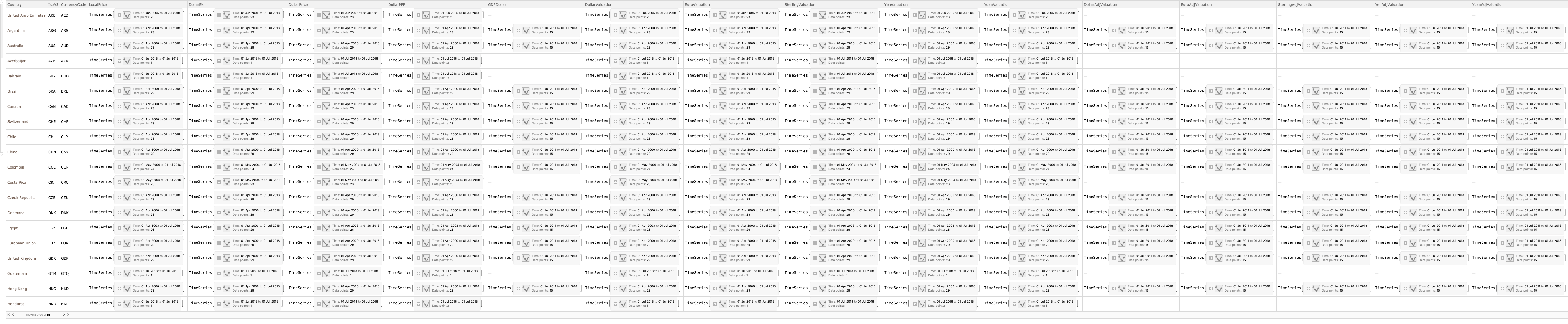Plot Dollar PPP against the Dollar Exchange rate for Argentina and China:

 In:=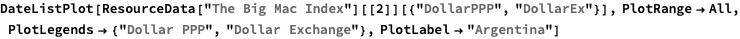Out=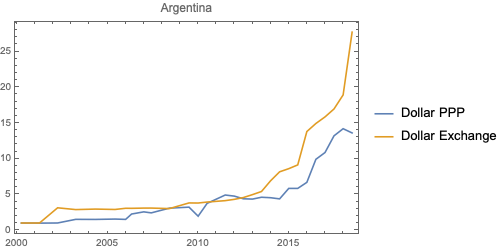In:=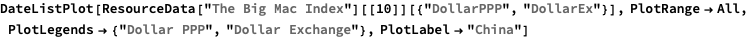Out=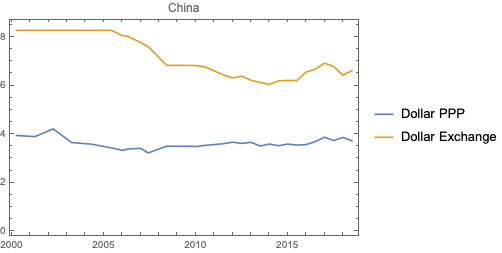Plot the changes in the Big Mac price in Chile relative to its US Dollar valuation:

 In:=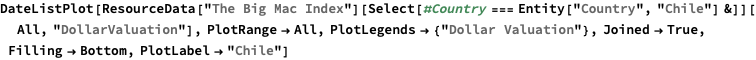Out=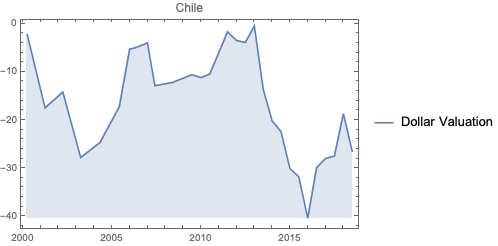Wolfram Research, "The Big Mac Index" from the Wolfram Data Repository (2018) https://doi.org/10.24097/wolfram.67673.data